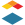# Community

cancel
Showing results for
Show  only  | Search instead for
Did you mean:Community Participant

## Do quiz formula question support greater than or equal >=

An instructor would like to use the formula below in quiz formula question.

if abs(zscore) > = 3

This results in an unrecognized token error. Can you use "> =" with the formula question? It would be helpful to know what functions are not supported in a formula question. Instructors want to use expressions that work in Excel, but from my experience Canvas is not as robust.

2 RepliesInstructure

Hello @lherdan

We might need further information on this. I was able to play around with the Equation editor in the RCE and I found that you can insert "> =" with the editor. The = option can be found on the basic area of the Equation editor. The > sign can be found on the Relationships screen in the editor.

I am not super familiar with using excel and how it makes the expressions in those programs.

I was able to find this GUIDE on using the Math Editor in the RCE.

HERE is a PDF on doing basic Equations.

HERE is a PDF on Advanced Equations.

HERE is a PDF on Chemistry formulas, equations, and scientific notationCommunity Participant

The RCE isn't available for the Formula Definition field. This is where you type the formula and where the instructor wants to use the symbols.

Thanks,

Linda